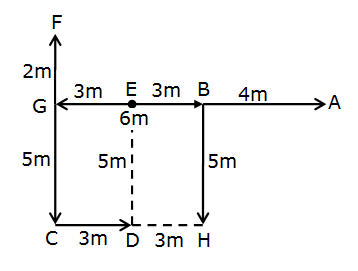# SBI Clerk Prelims Reasoning Ability Questions 2021 (Day-17)

Dear Aspirants, Our IBPS Guide team is providing new series of Reasoning Questions for SBI Clerk Prelims 2021 so the aspirants can practice it on a daily basis. These questions are framed by our skilled experts after understanding your needs thoroughly. Aspirants can practice these new series questions daily to familiarize with the exact exam pattern and make your preparation effective.

Start Quiz

Order and ranking

Directions (1-3): Study the following information carefully and answer the question below.

Seven persons P, Q, R, S, T, U and V are of different weights.

Q is lighter than T but heavier than S. R is heavier than Q and V. S is lighter than P but not as light as the lightest person. At least two persons are heavier than T. U is heavier than R but lighter than P.

1) Who is the 3rd-heaviest person?

A.T

B.Q

C.R

D.U

E.Cannot be determined

2) How many persons are lighter than Q?

A.Two

B.One

C.Three

D.Four

E.None of these

3) Who is the 2nd-heaviest person?

A.P

B.U

C.R

D.Q

E.T

Seating arrangement

Directions (4-8): Study the following information carefully and answer the question below.

P, Q, R, S, T, U, V and W are seated in a row and faces west direction but not necessarily in the same order.

R sits immediate right of U and 2ndto the left of P. Number of persons sits to the left of U is the same as the number of persons sits to the right of S. Only one person sits to the right of W. The one who sits immediate right of V, sits to the left of Q. T sits to the right of Q.

4) Who among the following sits immediate left of P?

A.V

B.Q

C.S

D.W

E.T

5) ___ and ____ are immediate neighbours of W?

A.Q and T

B.S and P

C.T and S

D.V and Q

E.P and V

6) What is the position of R with respect to Q?

A.Immediate left

B.2nd right

C.4th left

D.3rd left

E.4th right

7) How many persons sit towards the right of V?

A.5

B.7

C.4

D.3

E.6

8) T sits _____ of P?

A.Immediate left

B.2nd to the right

C.Immediate right

D.3rd to the left

E.4th to the left

Direction

Directions (9-10): Study the following information carefully and answer the question below.

Point A is 4m east of point B. Point C is 7m South of Point F and 3m west of Point D. Point E is at the midpoint of Point G and Point B which is 5m North of Point H. Point F is 2m North of Point G which is 10m west of Point A.

9) What is the shortest distance between point C and point H?

A.5m

B.6m

C.3m

D.4m

E.2m

10) What is the direction of point B with respect to point F?

A.South-East

B.North-West

C.West

D.North

E.North-East

Directions (1-3):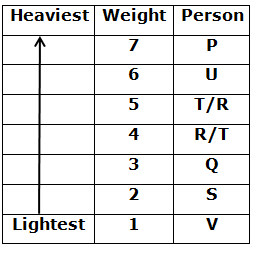Directions (4-8):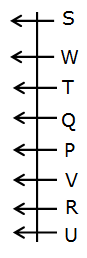R sits immediate right of U and 2ndto the left of P. Only one person sits to the right of W.

Here we get four possibilities.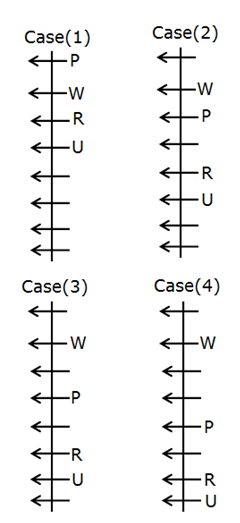Number of persons sits to the left of U is the same as the number of persons sits to the right of S.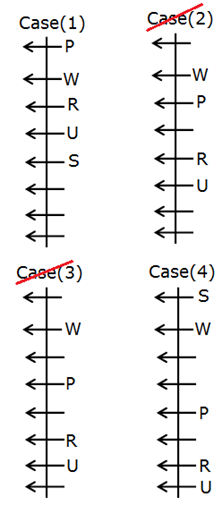We cannot fix the position of S in case (2) and case (3). So these cases are eliminated.

Person who sits immediate right of V is to the left of Q. T is to the right of Q.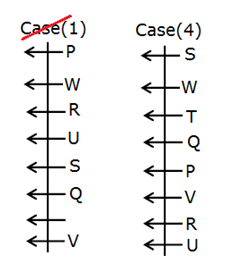We cannot fix the position of T in case (1). So this case is eliminated.

Directions (9-10):